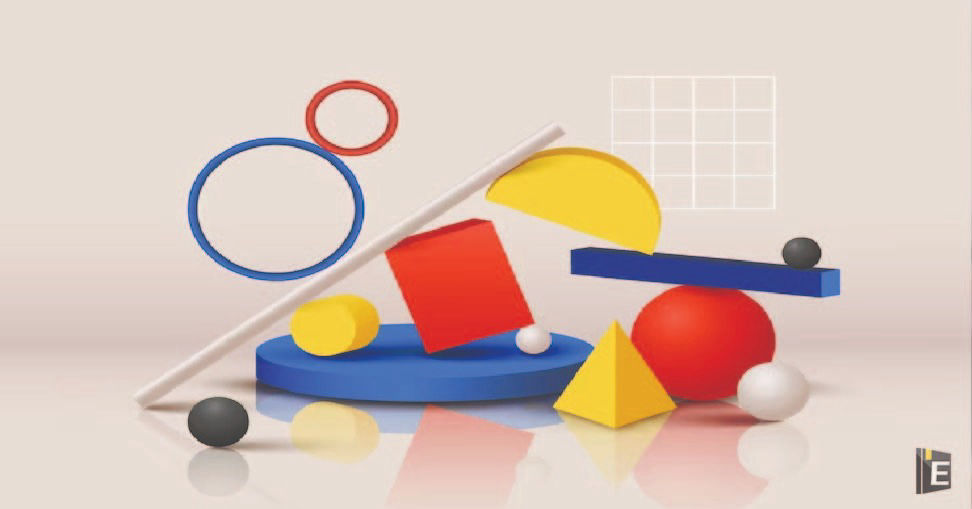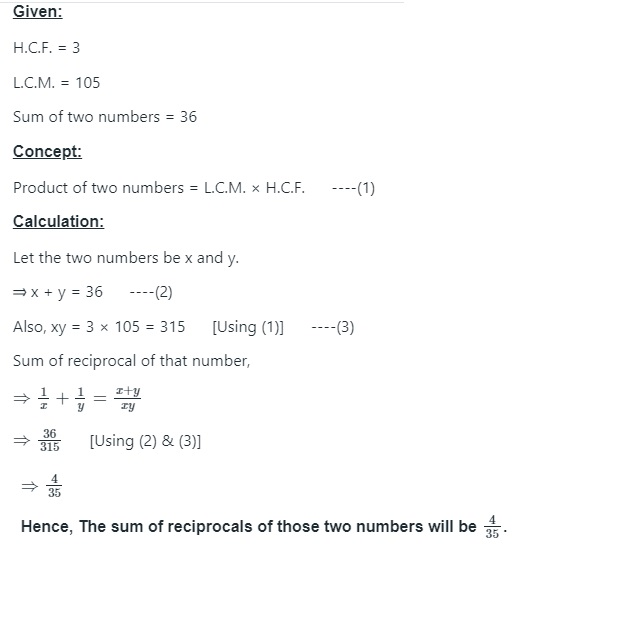• Save

# Mathematics Quiz Questions and Answers

NEWWelcome to our Mathematics Quiz Questions and Answers, where numbers come alive and equations challenge your mind! Prepare to delve into a world of calculations, patterns, and problem-solving. This Mathematics Quiz Questions and Answers quiz is designed to test your mathematical prowess and analytical thinking. From basic arithmetic to complex algebraic expressions, we've curated a collection of questions that will push your mathematical boundaries. Whether you're a math enthusiast or just looking to sharpen your skills, our quiz will provide an engaging and educational experience. Get ready to unravel the mysteries of numbers and unveil the solutions that lie within.

## Mathematics Quiz Questions

Here I am sharing Mathematics Quiz Questions and Answers related to Time and Distance, Time and Work, Average, HCF, LCM, Geometry, Mensuration etc for those learners who are preparing for upcoming competitive exams like SSC, Bank, RRB and other government exams. This Mathematics Quiz Questions and Answers blog will be helpful for you to crack any competitive exams.

Also, Read Latest Current Affairs Questions 2023: Current Affairs Today

"Stay ahead of the competition with our General Knowledge Mock Test and Current Affairs Mock Test!"

## Mathematics Quiz Questions and Answers

Q :

The sum of two numbers is 36 and their H.C.F and L.C.M. are 3 and 105 respectively. The sum of the reciprocals of two numbers is

(A) $${4\over 35}$$

(B) $${2\over 25}$$

(C) $${2\over 35}$$

(D) $${3\over 25}$$

Explanation :Q :

In △PQR, ∠P = 90°. S and T are the mid points of sides PR and PQ, respectively. What is the value of RQ2/(QS2+ RT2)?

(A) $$3\over 4$$

(B) $$4\over 5$$

(C) $$1\over 2$$

(D) $$2\over 3$$

Q :

A and B start moving from places X and Y and Y to X, respectively, at the same time on the same day. After crossing each other, A and B take $$5{4\over 9}$$ hours and 9 hours, respectively, to reach their respective destinations. If the speed of A is 33 km/h, then the speed (in km/h) of B is:

(A) 22

(B) 2

(C) $$25{2\over 3}$$

(D) $$24{1\over 3}$$

Q :

The sides of a triangle are in the ratio 2 : 3 : 4. The perimeter of the triangle is 18 cm. The area (in cm2) of the triangle is

(A) 9

(B) 36

(C) $$\sqrt {42}$$

(D) $$3\sqrt {15} sq.cm$$

Q :

Each side of an equilateral triangle is 6 cm. Find its area.

(A) 9 √3 sq.cm.

(B) 6 √3 sq.cm.

(C) 4√3sq.cm.

(D) 8 √3 sq.cm

Q :

If the numerical value of thes perimeter of an equilateral triangle is  $$\sqrt {3}$$ times the area of it, then the length of each side of the triangle is

(A) 2 units

(B) 3 units

(C) 4 units

(D) 6 units

Q :

The area of a right-angled isosceles triangle having hypotenuse 16√2 cm is

(A)

144 cm2

(B)

128 cm2

(C)

112 cm2

(D)

110 cm2

Q :

The area of two equilateral triangles are in the ratio 25 : 36. Their altitudes will be in the ratio :

(A) 36 : 25

(B) 25 : 36

(C) 5 : 6

(D) √5 : √6

Q :

From a point in the interior of an equilateral triangle, the length of the perpendiculars to the three sides are 6 cm, 8 cm and 10 cm respectively. The area of the triangle is

(A)

48 cm2

(B)

16√3 cm2

(C)

192√3 cm2

(D)

192 cm2

Q :

In an isosceles triangle, the measure of each of equal sides is 10 cm and the angle between them is 45°. the area of the triangle is

(A) $$25 \ cm^2$$

(B) $${25\over 2}\sqrt { 2}cm^2$$

(C) $$25 \sqrt { 2} \ cm^2$$

(D) $$25 \sqrt { 3} cm^2$$